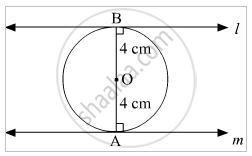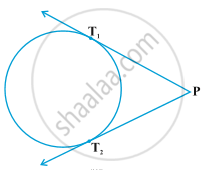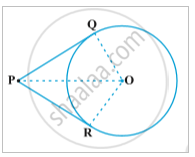# Number of Tangents from a Point on a Circle

#### description

Theorem - The Length of Two Tangent Segments Drawn from a Point Outside the Circle Are Equal

#### notes

A circle can have atmost two parallel tangents.l and m are two tangents, radii OA and OB are ⊥ m and l respectively. As studied in 9th class, if the sum of co-interior angles between two lines is 180° then the lines are parallel.

The point at which tangent touches a circle is known as point of contact. Here, point A and B are points of contact.

Only two tangents can be drawn from any external point of a circle.The length of the segment of the tangent from the external point P and the point of contact with the circle is called the length of the tangent from the point P to the circle.

The lengths PT1 and PT2 are equal, this we will learn in the further concepts.

#### theorem

Theorem- The lengths of tangents drawn from an external point to a circle are equal.Given: A circle C (o,r) and an external point P. PQ and PR are lengths of circle.

To prove: PQ=PR

Proof: In ΔPOQ and ΔPOR

PRO and PQO are right angled triangles (Theorem 1)

OQ = OR (Radii of the same circle)

Therefore, OQP ORP (Two right triangles are congruent if the hypotenuse and one side of one triangle are respectively equal to the hypotenuse and the corresponding side of the other triangle.)

This gives PQ = PR (CPCT)

If you would like to contribute notes or other learning material, please submit them using the button below.

### Shaalaa.com

Circles part 3 (Theorems Tangent Secant) [00:10:36]
S
0%